A particle of mass m is placed in the ground ...
A particle of mass m is placed in the ground state of a one- dimensional harmonic oscillator potential of the form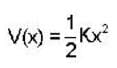where the stiffness constant K can be varied externally. The ground state wavefunction has the form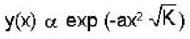where a is a constant. If suddenly, the parameter k is changed to 4K, the probability that the particle will remain in the ground state of new potential is _____ .
A particle of mass m is placed in the ground state of a one- dimension...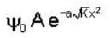where A is normalisation constant
when parameter K is suddenly changed to 4K, the wave function associated with ground state of new potential is given by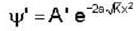Probability that particle will be found in ground state p =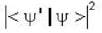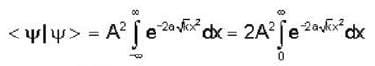Put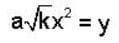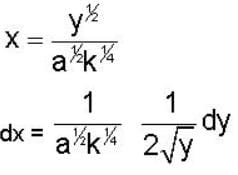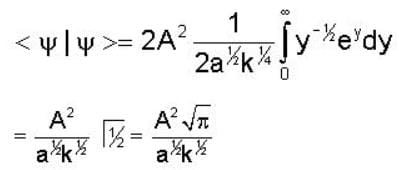From Normalisation equation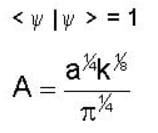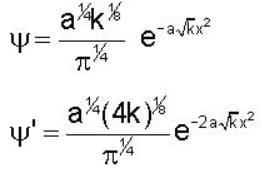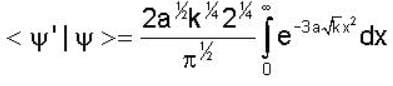Let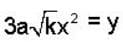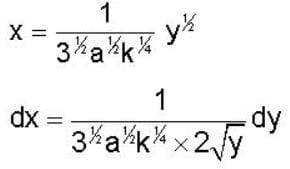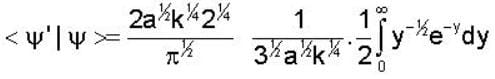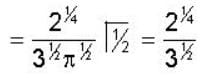Probability P =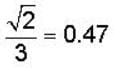1 Crore+ students have signed up on EduRev. Have you?
1 Crore+ students have signed up on EduRev. Have you?

### Learn this topic in detailGATE Physics Mock Test Series - 2 60 Ques | 180 MinsView courses related to this question

### Quick links for GATE exam850+
Video Lectures2500+
Revision Notes600+
Online Tests34+
Courses
A particle of mass m is placed in the ground state of a one- dimensional harmonic oscillator potential of the formwhere the stiffness constant K can be varied externally. The ground state wavefunction has the formwhere a is a constant. If suddenly, the parameter k is changed to 4K, the probability that the particle will remain in the ground state of new potential is _____ .Correct answer is '0.47'. Can you explain this answer?
Question Description
A particle of mass m is placed in the ground state of a one- dimensional harmonic oscillator potential of the formwhere the stiffness constant K can be varied externally. The ground state wavefunction has the formwhere a is a constant. If suddenly, the parameter k is changed to 4K, the probability that the particle will remain in the ground state of new potential is _____ .Correct answer is '0.47'. Can you explain this answer? for GATE 2023 is part of GATE preparation. The Question and answers have been prepared according to the GATE exam syllabus. Information about A particle of mass m is placed in the ground state of a one- dimensional harmonic oscillator potential of the formwhere the stiffness constant K can be varied externally. The ground state wavefunction has the formwhere a is a constant. If suddenly, the parameter k is changed to 4K, the probability that the particle will remain in the ground state of new potential is _____ .Correct answer is '0.47'. Can you explain this answer? covers all topics & solutions for GATE 2023 Exam. Find important definitions, questions, meanings, examples, exercises and tests below for A particle of mass m is placed in the ground state of a one- dimensional harmonic oscillator potential of the formwhere the stiffness constant K can be varied externally. The ground state wavefunction has the formwhere a is a constant. If suddenly, the parameter k is changed to 4K, the probability that the particle will remain in the ground state of new potential is _____ .Correct answer is '0.47'. Can you explain this answer?.
Solutions for A particle of mass m is placed in the ground state of a one- dimensional harmonic oscillator potential of the formwhere the stiffness constant K can be varied externally. The ground state wavefunction has the formwhere a is a constant. If suddenly, the parameter k is changed to 4K, the probability that the particle will remain in the ground state of new potential is _____ .Correct answer is '0.47'. Can you explain this answer? in English & in Hindi are available as part of our courses for GATE. Download more important topics, notes, lectures and mock test series for GATE Exam by signing up for free.
Here you can find the meaning of A particle of mass m is placed in the ground state of a one- dimensional harmonic oscillator potential of the formwhere the stiffness constant K can be varied externally. The ground state wavefunction has the formwhere a is a constant. If suddenly, the parameter k is changed to 4K, the probability that the particle will remain in the ground state of new potential is _____ .Correct answer is '0.47'. Can you explain this answer? defined & explained in the simplest way possible. Besides giving the explanation of A particle of mass m is placed in the ground state of a one- dimensional harmonic oscillator potential of the formwhere the stiffness constant K can be varied externally. The ground state wavefunction has the formwhere a is a constant. If suddenly, the parameter k is changed to 4K, the probability that the particle will remain in the ground state of new potential is _____ .Correct answer is '0.47'. Can you explain this answer?, a detailed solution for A particle of mass m is placed in the ground state of a one- dimensional harmonic oscillator potential of the formwhere the stiffness constant K can be varied externally. The ground state wavefunction has the formwhere a is a constant. If suddenly, the parameter k is changed to 4K, the probability that the particle will remain in the ground state of new potential is _____ .Correct answer is '0.47'. Can you explain this answer? has been provided alongside types of A particle of mass m is placed in the ground state of a one- dimensional harmonic oscillator potential of the formwhere the stiffness constant K can be varied externally. The ground state wavefunction has the formwhere a is a constant. If suddenly, the parameter k is changed to 4K, the probability that the particle will remain in the ground state of new potential is _____ .Correct answer is '0.47'. Can you explain this answer? theory, EduRev gives you an ample number of questions to practice A particle of mass m is placed in the ground state of a one- dimensional harmonic oscillator potential of the formwhere the stiffness constant K can be varied externally. The ground state wavefunction has the formwhere a is a constant. If suddenly, the parameter k is changed to 4K, the probability that the particle will remain in the ground state of new potential is _____ .Correct answer is '0.47'. Can you explain this answer? tests, examples and also practice GATE tests.(Scan QR code)